# Math

You are currently browsing the archive for the Math category.

## Fibonacci Fractions (Part 2) – A fairly quick derivation

Yesterday, I wrote about how the fractions

\begin{aligned} \frac{1000}{998999} &= 0.00100100200300500801302103405509… \\ \frac{10000}{99989999} &= 0.000100010002000300050008001300210… \\ \frac{100000}{9999899999} &= 0.0000100001000020000300005000080001… \\ \frac{1000000}{999998999999} &= 1.000001000002000003000005000008000013…\cdot 10^{-6} \\ \frac{10000000}{99999989999999} &= 1.000000100000020000003000000500000080000013\cdot 10^{-7} \\ \end{aligned}

display the first several values of the Fibonacci sequence.  The general formula for these fractions is $$f_k = \frac{10^k}{10^{2 k} – 10^k – 1}.$$

Today, I will provide a fairly simple derivation for this formula without summing an infinite series.

Let $a_1=1, a_2=1, a_3=2, a_4=3, a_5=5, a_6=8,\ldots$ be the Fibonacci sequence.  Let $a_i=0$ when $i\leq 0$.  Now, we know that by definition

$$a_n = a_{n-1} + a_{n-2}$$

for all $n>1$.  The formula above also holds for $n<1$, but fails for $n=1$.  We can fix that by adding in the Kronecker delta function $\delta_{i,j}$ which is 0 if $i\neq j$ and 1 when $i=j$.  The amended formula valid for all $n$ is

$$a_n = a_{n-1} + a_{n-2} +\delta_{n,1}.$$

In the prior article, we defined the Fibonacci fractions to be

$$f_k = \sum_{i=1}^\infty \frac{a_i}{10^{\ ki}}.$$

So,

\begin{aligned} f_k &= \sum_{i=1}^\infty \frac{a_i}{10^{\ ki}} \\ &= \sum_{i=1}^\infty \frac{a_{i-1} + a_{i-2} + \delta_{i,1}}{10^{\ ki}}\\&= \sum_{i=1}^\infty \frac{a_{i-1}}{10^{\ ki}} +\frac{a_{i-2}}{10^{\ ki}} + \frac{\delta_{i,1}}{10^{\ ki}}\\&= \frac{1}{10^{\ k}} + \sum_{i=1}^\infty \frac{a_{i-1}}{10^k10^{\ k(i-1)}} +\frac{a_{i-2}}{10^{2k}10^{\ k(i-2)}} \\&= \frac{1}{10^{\ k}} + \frac{f_k}{10^k} +\frac{f_k}{10^{2k}}. \end{aligned}

Thus,

$$f_k= \frac{1}{10^{\ k}} + \frac{f_k}{10^k} +\frac{f_k}{10^{2k}}$$

$$f_k – \frac{f_k}{10^k} -\frac{f_k}{10^{2k}} = \frac{1}{10^{\ k}}$$

$$f_k \left(1 – \frac{1}{10^k} -\frac{1}{10^{2k}}\right) = \frac{1}{10^{\ k}}$$

$$f_k = \frac{\frac{1}{10^{\ k}}}{ 1 – \frac{1}{10^k} -\frac{1}{10^{2k}} }$$

$$f_k = \frac{10^{\ k}}{ 10^{2k} – 10^k -1 }$$

which completes the derivation.

This technique is often used for Z-transforms and generating functions.

## Fibonacci Fractions

I think that it is really cool that some fractions have interesting patterns in their decimal expansion.  For example,

$$1/499 = 0.0020040080160320641282565130260….$$

You can see the first 8 values of the doubling sequence (a.k.a the powers of 2)  2, 4, 8, 16, 32, 64, 128, and 256 in the decimal expansion.  If you want more values, you can just add more 9’s to the denominator.

\begin{aligned} 1/49999 = &0.0000200004000080001600032000640012800256005120\\&1024020480409608192163\ 8432768655373107462….\end{aligned}

Other fractions can encode other sequences.  The first several square numbers 1, 4, 9, 16, … are encoded by the fractions

$$\small\frac{10100}{970299},\frac{1001000}{997002999},\frac{100010000}{999700029999},\frac{10000100000}{999970000299999},\frac{1000001000000}{999997000002999999},….$$

For example,

$$\frac{1001000}{997002999} = 0.001004009016025036049064081100121144169196225….$$

It turns out that it is not too hard to find sequences of fractions which encode any sequence of positive integers that is a sum of

• polynomials  (e.g.  cubes         1, 8, 27, 64, 125, …),
• exponentials (e.g. powers of 3  1, 3, 9, 27, 81, 243, …), or
• products of polynomials and exponentials.  (e.g.  cubes times power of 3     1, 24, 243, 1728, 10125, 52488, 250047,…).

### The Fibonacci sequence

The Fibonacci sequence is

1, 1, 2, 3, 5, 8, 13, 21, 34, 55, 89, 144, 233, 377, 610, 987, 1597, 2584, 4181, 6765, ….

Each value after the initial ones is the sum of the previous two values (e.g. 2 +3 =5, 3+5 =8, …).  It turns out that the Fibonacci sequence is the sum of exponentials, so it also can be encoded in a sequence of fractions.

\begin{aligned} \frac{1000}{998999} &= 0.00100100200300500801302103405509… \\ \frac{10000}{99989999} &= 0.000100010002000300050008001300210… \\ \frac{100000}{9999899999} &= 0.0000100001000020000300005000080001… \\ \frac{1000000}{999998999999} &= 1.000001000002000003000005000008000013…\cdot 10^{-6} \\ \frac{10000000}{99999989999999} &= 1.000000100000020000003000000500000080000013\cdot 10^{-7} \\ \end{aligned}.

All of these fractions $f_k$ can be computed with the formula

$$f_k=\sum_{i=1}^\infty \frac{a_i}{10^{\ k i}}$$

where the $a_i$ are the values of the sequence and $k$ is a positive integer specifying the spacing between the values of $a$ in the decimal expansion.   This sum is fairly easy to compute when $a$ is a polynomial, an exponential, or a combination of polynomials and exponentials.

For example, to get the doubling numbers $a_i = 2^i$, the fractions would be

\begin{aligned}\sum_{i=1}^\infty \frac{a_i}{10^{\ k i}}&= \sum_{i=1}^\infty \frac{2^i}{10^{\ k i}}\\&= \sum_{i=1}^\infty \left(\frac{2}{10^k}\right)^i\\&=\frac{\frac{2}{10^k}}{1-\frac{2}{10^k}}\quad{\text{using} \sum_{i=1}^\infty x^i= \frac{x}{1-x}}\\&= \frac{2}{10^k – 2}.\end{aligned}

For the Fibonacci sequence $$a_i = \frac{\varphi^i-\psi^i}{\varphi-\psi}= \frac{\varphi^n-\psi^n}{\sqrt 5}$$ where $$\varphi = \frac{1 + \sqrt{5}}{2} \approx 1.61803$$ and $$\psi = \frac{1 – \sqrt{5}}{2} = 1 – \varphi = – {1 \over \varphi} \approx -0.61803.$$ (Copied verbatim with glee from the Wikipedia).

The math required to compute the Fibonacci fractions is almost the same as the powers of two math using the same $$\sum_{i=1}^\infty x^i= \frac{x}{1-x}$$ trick.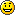After you go through the computations, the Fibonacci fractions can be found with the formula

$$f_k = \frac{10^k}{10^{2 k} – 10^k – 1}.$$

## Decimal Expansion of 1/7

Most people look at the first four digits of 1/7 ~= .1428 and think that the fact that 7*2=14 and 7*4=28 is a mere coincidence. It’s not. Let’s compute the decimal expansion of 1/7 without doing long division, but using instead $$x/(1-x) = x + x^2 + x^3 + \ldots.$$
1/7
= 7/49
= 7*1/(50-1)
= 7*1/50/(1-1/50)
= 7*.02/(1-.02)
= 7*(.02 + .02^2 + .02^3 + …)
= 7*(.02 + .0004 + .000008 + .00000016 + .0000000032 + …)
= 7*(.020408163264…)
= 7*(.020408) + 7*(..000000163264)
= .142856 + 7*(.00000016) + 7*(.00000000326…)
= .142856 + .00000112 + 7*(.00000000326…)
Notice that the first n digits must be .142857. The decimal expansion of 1/j either terminates or repeats with at most (j-1) repeating digits and we have 6 digits, so those six digits must repeat, thus
1/7 = .142857142857142857142857142857142857….

## A proof of Griffin’s Blackjack Theorem

Professor Peter Griffin discovered a nice theorem about the best way to count cards in Blackjack. (See the appendix in his book “Theory of Blackjack”). In this note, we review the theorem and reproduce his proof with more detail.

Suppose that you have a wagering game like blackjack which is played with a deck of cards. If you remove some cards from the deck, then the expected value of the game changes. Griffin found an easy formula for estimating the change in expectation caused by the removal of the cards. His formula depends on $E_j$ which is the change in expectation of the game when removing only the $j$th card from the deck.

Assume that the game never requires more than $k$ cards and the deck has $n$ cards in it. Obviously $n\geq k>0$. There are $m = {n \choose k}$ subsets of the deck $I_1, I_2, \ldots, I_m$ that contain $k$ cards each. Let $y_i$ be the expected value of the game played with the card subset $I_i$. We would like to estimate $y_i$ based on the cards in $I_i$.

We can create a linear estimate of $y_i$ by creating a vector $b=(b_1, b_2, \ldots, b_n)$ where $b_j$ is the “value” of the $j$th card. More specifically,
$$y_i \approx \sum_{j\in I_i} b_j.$$
Griffin found that the correct formula for $b_j$ is simply $$b_j = (\mu – (n-1) E_j)/k$$ where $\mu$ is the expected value of the game with a fresh deck. Using this value vector $b$ minimizes the squared error of the estimator.  This formula is remarkable both for its simplicity and the fact that $k$ only plays a small roll in the calculation.

Griffin’s Theorem Let the error function $e:R^m \rightarrow R$ be defined as

$$e(b) = \sum_{i=1}^m \left( y_i – \sum_{j\in I_i} b_j \right)^2 .$$
Then $e(b^*) = \min_{b\in R^m} e(b)$ is the unique global minimum of $e$ where
$$b^*_j = (\mu – (n-1) E_j)/k$$
and $\mu$ is the expected value of the game with a fresh deck.

In the theorem above, $e$ is the sum of the squared errors in the linear estimate of the expected value of the game. In order to prove the theorem, we need two lemmas.

Lemma 1 If $\tilde{y}_j$ is the average expectation of the $k$ card subsets that do not contain card $j$, $\bar{y}_j$ is the average expectation of the $k$ card subsets that contain card $j$, and $\mu$ is the expectation of the game with a fresh deck, then
$$\mu = \frac{k}{n}\; \bar{y}_j + \left(1- \frac{k}{n}\right)\tilde{y}_j$$
and
$$\bar{y}_j = \frac{n \mu – (n-k) \tilde{y}_j}{k}.$$

The short proof of this lemma is left to the reader.

Lemma 2
Suppose for $j = 1,\ldots, n$,
$$(2.1)\quad b_j + \alpha \sum_{i=1}^n b_i = \gamma_j.$$  Then

$$b_j = \gamma_j – \frac{\alpha\; n\; \bar\gamma}{1 + n \alpha}$$
where $\bar\gamma = \frac1n \sum_{j=1}^n \gamma_j$.

Proof: Sum both sides of equation (2.1) to get
$$n \bar{b} + \alpha n^2 \bar{b} = n \bar{\gamma}$$
where $\bar{b} = \frac1n \sum_{j=1}^n b_j$. Then,
\begin{aligned} \bar{b} + \alpha n \bar{b} &= \bar{\gamma} \\ \bar{b} &= \frac{\bar{\gamma}}{1+ n \alpha}. \end{aligned}
Applying that to equation (2.1) yields
\begin{aligned} b_j + \alpha \sum_{j=1}^n b_j &= \gamma_j \\ b_j + \alpha n \bar{b} &= \gamma_j\\ b_j + \alpha n \frac{\bar{\gamma}}{1+n \alpha} &= \gamma_j\\ b_j &= \gamma_j – \frac{\alpha\; n\; \bar{\gamma}}{1+n \alpha} \\ \end{aligned}
which completes the proof of the Lemma.

Now we can prove Griffin’s Theorem.

Proof:  Let the matrix $X$ be defined by $X_{ij} = 1$ if card $j$ is in set $I_i$ and $X_{ij}=0$ otherwise. We wish to minimize the sum of the squared errors. If we assume that the value of the $j$th card is $b_j$, then we can estimate the expectation of the game played using only card subset $I_i$ with
$$\sum_{j\in I_i} b_j.$$
The error of this estimate is $(y_i – \sum_{j\in I_i} b_j)$. The sum of the squared error is
$$e(b) = \sum_{i=1}^m \left( y_i – \sum_{j\in I_i} b_j \right)^2.$$
Noice that $\sum_{j\in I_i} b_j = x_i \cdot b$ where $x_i$ is the $ith$ row of $X$. So,
$$e(b) = \sum_{i=1}^m \left( y_i – \sum_{j=1}^n X_{ij} b_j \right)^2 = \| X b – y \|^2.$$
In other words $e(b)$ is the square of the distance between $y$ and $Xb$. The Gauss-Markov theorem provides a nice solution for the $b$ which minimizes this distance
$$b^* = \left(X^T X\right)^{-1} X^T y$$
where $X^T$ indicates the transpose of $X$. Hence,
$$(1)\quad X^T X b^* = X^T y.$$
Let $C=X^T X$. It turns out that $C$ has a very simple structure.

$C_{ij} = x_i^T x_j$ where $x_i$ and $x_j$ are the $i$th and $j$th columns of $X$, so
$$(2) \quad C_{ii} = x_i^T x_i = { n-1 \choose k-1},$$
and if $i \neq j$,
$$(3)\quad C_{ij} = x_i^T x_j = { n-2 \choose k-2}.$$

So, applying equations (2) and (3) to  $i$th row of matrix equation (1) gives

$${n-1 \choose k-1} b_i^* + {n-2 \choose k-2} \sum_{j\neq i} b_j^* = {n-1 \choose k-1} {\bar{y}_i}$$
for $j=1, 2, \ldots n$ where $\bar{y}_j$ is the average expectation of the ${n-1\choose k-1}$ subsets with $k$ cards that contain card $j$.

Note that $${n-2 \choose k-2} / {n-1 \choose k-1} = \frac{k-1}{n-1} ,$$ so
\begin{aligned} b_i^* + \frac{k-1}{n-1} \sum_{j\neq i}^n b_j^* &= \bar{y}_i \\ b_i^* – \frac{k-1}{n-1} b_i^* + \frac{k-1}{n-1} \sum_{j=1}^n b_j^* &=\bar{y}_i \\ \frac{n-k}{n-1} b_i^* + \frac{k-1}{n-1} \sum_{j=1}^n b_j^* &= \bar{y}_i \\ (n-k) b_i^* + (k-1) \sum_{j=1}^n b_j^* &= (n-1) \bar{y}_i\\ b_i^* + \frac{k-1}{n-k} \sum_{j=1}^n b_j^* &= \frac{n-1}{n-k}\bar{y}_i. \end{aligned}
We apply Lemma 2 with $\alpha = \frac{k-1}{n-k}$ and $\gamma_j = \frac{n-1}{n-k} \bar{y}_j$ to get
\begin{aligned} b_j^* &= \gamma_j – \frac{\alpha\; n\; \bar\gamma}{1 + n \alpha} \\ &= \frac{n-1}{n-k} \bar{y}_j\; – \frac{\frac{k-1}{n-k}\; n\; \bar\gamma}{1 + n\; \frac{k-1}{n-k}} \\ &= \frac{n-1}{n-k} \bar{y}_j\; – \frac{(k-1) \; n\; \bar\gamma}{n-k + n(k-1)} \\ &= \frac{n-1}{n-k} \bar{y}_j\; – \frac{(k-1) \; n\; \bar\gamma}{n-k + n k-n} \\ &= \frac{n-1}{n-k} \bar{y}_j\; – \frac{(k-1) \; n\; \bar\gamma}{-k + n k} \\ &= \frac{n-1}{n-k} \bar{y}_j\; – \frac{(k-1) \; n\; \bar\gamma}{k (n-1)} \\ &= \frac{n-1}{n-k} \bar{y}_j\; – \frac{(k-1) \; n\; \frac{n-1}{n-k} \mu }{k (n-1)} \\ &= \frac{n-1}{n-k} \bar{y}_j\; – \frac{(k-1) \; n\; \mu }{(n-k)k}. \\ \end{aligned}
By Lemma 1,
$$\bar{y}_j = \frac{n \mu – (n-k) \tilde{y}_j}{k},$$
so
\begin{aligned} b_j^* &= \frac{n \mu – (n-k) \tilde{y}_j}{k} \frac{n-1}{n-k} – \frac{ n (k-1)\mu}{k (n-k)} \\ &= \frac{ n (n-1) \mu }{(n-k)k} – \frac{ (n-k) \tilde{y}_j (n-1)}{k(n-k)} – \frac{ n (k-1)\mu}{k (n-k)} \\ &= \frac{ n (n-1) \mu }{(n-k)k} – \frac{ n (k-1)\mu}{k (n-k)} – \frac{ (n-k) \tilde{y}_j (n-1)}{k(n-k)} \\ &= \frac{n \mu}{k} \left[ \frac{n-1}{n-k} - \frac{k-1}{n-k} \right] – \frac{ \tilde{y}_j (n-1)}{k} \\ &= \frac{n \mu}{k} – \frac{ \tilde{y}_j (n-1)}{k} \\ &= \frac{n \mu – (n-1) \tilde{y}_j}{k} \\ &= \frac{\mu – (n-1) (\tilde{y}_j- \mu)}{k} \\ &= \frac{\mu – (n-1) E_j}{ k } \end{aligned}
which completes the proof.

In the future, I hope to write some articles about how Griffin’s Theorem can be applied to other games.

I should mention that it is not too hard to extend Griffin’s Theorem to get more accurate quadratic approximation of the expectation of the game with card removal (or higher degree polynomial approximations).

## Visualizing Cramer’s Rule

I posted the comment below on Reddit.

It easy for me to recall the geometric picture Cramer’s rule in my head, but it took an hour or more to write down an explanation.

Suppose that the vector $y = a_1 x_1 + a_2 x_2 + \cdots + a_n x_n$
where $y, x_1, x_2, \ldots, x_n$ are all vectors in $R^n$ and $a_1,a_2, \ldots, a_n$ are real.

(We will assume that $\det(x_1,x_2, \ldots, x_n)$ is not zero.)
For me, Cramer’s rule is just two hyperparallelepiped (squished hypercubes) which share the same base.

The first “parallelepiped” has edges $a_1 x_1, a_2 x_2, a_2 x_3,\ldots, a_nx_n$.
The second “parallelepiped” has edges $y, a_2 x_2, a_2 x_3,\ldots, a_n x_n.$
The shared base has edges $a_2 x_2, a_3 x_3,\ldots, a_n x_n$.

The volume of the first “parallelepiped” is $\det(a_1 x_1, a_2 x_2, \ldots, a_n x_n)$.
The volume of the second “parallelepiped” is $\det(y, a_2 x_2, \ldots, a_n x_n)$.
There are two key insights:

1) these parallelepipeds have the same “height” and base, and hence the same volume.
2) the ratio of the volumes is 1, so

1 = “volume of parallelepiped 1″/”volume of parallelepiped 2″ (by insight 1)
= $\frac{\det(a_1 x_1, a_2 x_2, \ldots, a_n x_n)}{\det(y, a_2 x_2, a_3 x_3, \ldots, a_n x_n)}$
= $a_1 \frac{\det(x_1, x_2, \ldots, x_n)}{\det(y, x_2, x_3, \ldots, x_n)}$ (by linearity of the determinant).

The second insight leads directly to Cramer’s rule by multiplying both sides of the equality by
$$\frac{\det(y, x_2, \ldots, x_n)}{\det(x_1, x_2, x_3, \ldots, x_n)}.$$

Why is the first insight true?

To explain this
let B= span(base) = $span(x_2,x_3, \ldots, x_n)$ and
let A= the one dimensional subspace perpendicular to A.

Think of the surface of a table as being B. The height of each parallelepiped is the distance from B to the points in the parallelepiped which are farthest away from B.
The height of the first cube, $h_1$, is the distance from the base which lies in B to the “top” of the parallelepiped 1 which is parrallel to B.
$$h_1 = proj_A(a_1 x_1) = a_1 proj_A(x_1).$$

The height of the second cube, $h_2$, is the distance from the base which lies in B to “top” of the parallelepiped 2 which is parrallel to B.
$$h_2 = proj_A(y).$$

But, by the defintion of y and the fact that A is perpendicular to $x_2,x_3, \ldots, x_n,$
\begin{aligned} h_2 &= proj_A(y)\\ &= proj_A(a_1 x_1+a_2 x_2+\cdots+a_n x_n) \\ &= proj_A(a_1 x_1) + proj_A(a_2 x_2+\cdots+a_n x_n) \\ &= proj_A(a_1 x_1) \\ &= a_1 proj(x_1) \\ &= h_1. \end{aligned}
This shows that the “heights of the two parallellepipeds are equal, so the volumes must be equal because they share the same base.

———————————————

Here is almost same idea with a lot fewer words.
Assume without loss of generality that the space is $span(x_1,x_2, \ldots, x_n)$

Let B = $span( x_2, x_3, \ldots, x_n)$.
Let A = the 1 dimensional subspace which is perpendicular to A.
Then
\begin{aligned} proj_A y &= proj_A (a_1 x_1+ \cdots + a_n x_n) \\ &= proj_A (a_1 x_1) \\ &\mathrm{\ \ \ \ (due\ to\ linearity\ and\ the\ fact\ that\ B\ is\ perpendicular\ to\ } x_2,x_3,\ldots, \mathrm{\ and\ } x_n)\\ &= a_1 proj_A(x_1). \end{aligned}

So, $|a_1| = \frac{length( proj_A\; y)}{ length( proj_A\; x_1)}.$

Let
$\alpha = |\det(x_1,x_2,\ldots, x_n)|$ = “volume of the parallelepiped with edges $x_1, x_2, x_3, \ldots, x_n$”,
$\beta = |\det(y, x_2,\ldots, x_n)|$ = “volume of the parallelepiped with edges $y, x_2, x_3, \ldots, x_n$”, and
$\gamma =$ “area of the hyperrhombus with edges $x_2, x_3, \ldots, x_n$”.

$\alpha = length( proj_A x_1)\; \gamma$
$\beta = length( proj_A y)\; \gamma$

Finally,
\begin{aligned} |a_1| &= \frac{length( proj_A y) }{ length( proj_A x_1)} \\ &= \frac{|length( proj_A y) \gamma| }{ | length( proj_A x_1) \gamma) |} \\ &=\frac{ |\det(y, x_2, \ldots, x_n)| }{ |\det(x_1, x_2, \ldots x_n)|}. \end{aligned}

## One reason why I want 30 digits of Pi or even more

I was wondering how fast the Taylor Series for $\exp(\pi i)$ would converge.  According to Taylor,

$$\exp(\pi i) = 1 + (\pi i) + (\pi i)^2/2! + (\pi i)^3/3! + (\pi i)^4/4! + \ldots = \sum_{j=0}^\infty \frac{(\pi i)^j}{j!}.$$

According to Euler, $\exp(\pi i)= -1$.  I wanted to estimate the convergence rate, so I just fired up Mathematica and plotted the values of $$error(k) = \left| 1+ \sum_{j=0}^\infty \frac{(\pi i)^j}{j!}\right|.$$  The Mathematica plot is shown below.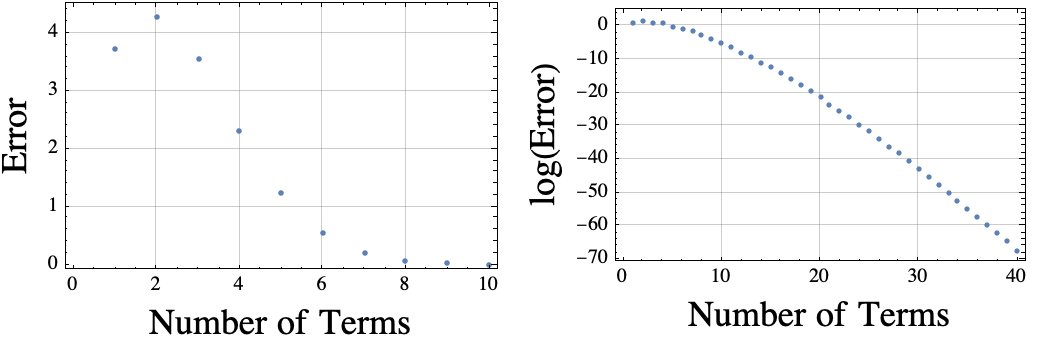It occurred to me that Mathematica knows thousands of digits of pi.  I could force it to only use the first 16 digits by using double precision floating point numbers. If I do that, then the log(Error) plot is “wrong” because the approximation for pi is not accurate enough.  The erroneous plot is shown below.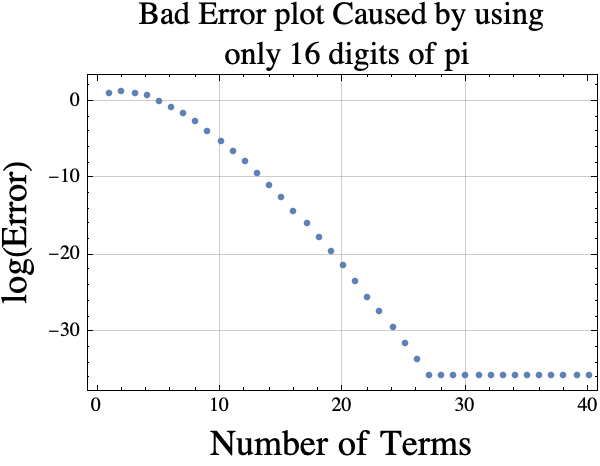If you want a good estimate of the truncated Taylor series error using $k$ terms, you could use the absolute value of term $k+1$ which is

$$error(k)\approx f(k)= \frac{\pi^{k+1}}{(k+1)!}$$.

Here is a plot of $r(k)= error(k)/f(k)$.  If the estimator is good, then the $r(k)$ values (y-coordinates) should be near 1.Creating these plots requires around 30 digits of pi!  If I wanted to look at more terms, I would need more digits of pi.

Here is the code I used to generate the plots.

SetOptions[ListPlot, GridLines -> Automatic, Frame -> True, ImageSize -> 250];
error[k_] := Abs[ 1 + Sum[ (I Pi)^j/j!, {j, 0, k}]];
plt0 = ListPlot[ Array[error, 10], Frame -> True,
FrameLabel -> (Style[#, 18] & /@ {"Number of Terms", "Error"})];
plt1 = ListPlot[Log[ Array[error, 40]],
FrameLabel -> (Style[#, 18] & /@ {"Number of Terms",
"log(Error)"})];
error[k_] := Abs[ 1 + Sum[ (I N[Pi])^j/j!, {j, 0, k}]];
plt3 = ListPlot[Log[ Array[error, 40]], Frame -> True,
FrameLabel -> (Style[#, 18] & /@ {"Number of Terms", "log(Error)",
"Bad Error plot Caused by using only 16 digits of pi", " "}),
PlotLabel ->
Style["Bad Error plot Caused by using\n only 16 digits of pi",
16]];
errorRat[k_] :=
Abs[ 1 + Sum[ (I Pi)^j/j!, {j, 0, k}]]/ (Pi^(k + 1)/(k + 1)! );
plt4 = ListPlot[Array[errorRat, 40], Frame -> True,
FrameLabel -> (Style[#, 18] & /@ {"Number of Terms",
"error(k)/f(k)"}),
PlotLabel ->
Style["Plot error(k) divided by estimated\n error(k) for \
k=1,2,..., 40", 16]];

## Maximizing a function over integers (Part 3)

In part 1, we defined the term strictly concave and introduced the theorem that if $f$ is strictly concave and $f(x)=f(x+1)$, then the integer(s) that maximize $f$ are exactly the set $\{\mathrm{floor}(x+1), \mathrm{ceil}(x)\}$ which is usually a set containing only one number $\mathrm{round}(x+1/2)$.  In part 2, we showed how the theorem in part 1 can be used to prove that the maximum value that can be obtained when putting an integer into $f(x)=a x^2 + b x + c$ is $f(i)$ where $i=\mathrm{round}(-b/(2a))$ (assuming $a<0$).

The next theorem says that the real number $x$ that maximizes $f$ is always near the integer $i$ that maximizes $f$.

### THEOREM #3

If

• $f$ is a real valued strictly concave function,
• there is a real number $x$ such that $f(x)$ is the maximum value that $f$ attains with real inputs, and
• there is an integer $i$ such that $f(i)$ is the maximum value that $f$ attains with integer inputs,

then $|x-i|<1$.

The idea of the proof is fairly simple.  Suppose that $i>x+1$.  Then $i-1>x$. So $i-1$ is between $i$ and $x$.  Concavity implies that that the point $(i-1, f(i-1))$ lies above the line connecting $(x, f(x))$ and $(i,f(i))$, but $f(x)\geq f(i)$.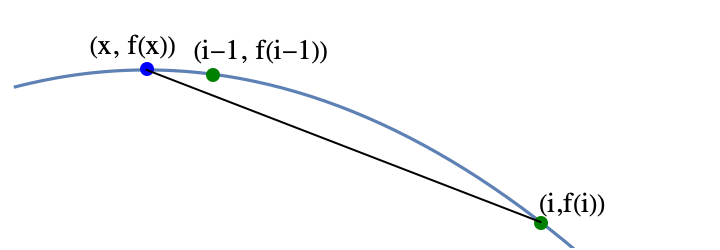The fact that the point $(i-1, f(i-1))$ is above the line implies that $f(i-1)> f(i)$, but that contradicts the definition of $i$, so using proof by contradiction, $i\leq x+1$.  The other cases are either similar or easy.

### EXAMPLE

Find the maximum value of  $f(x) = x (x-14) (x-20)$ among all integers between 0 and 14.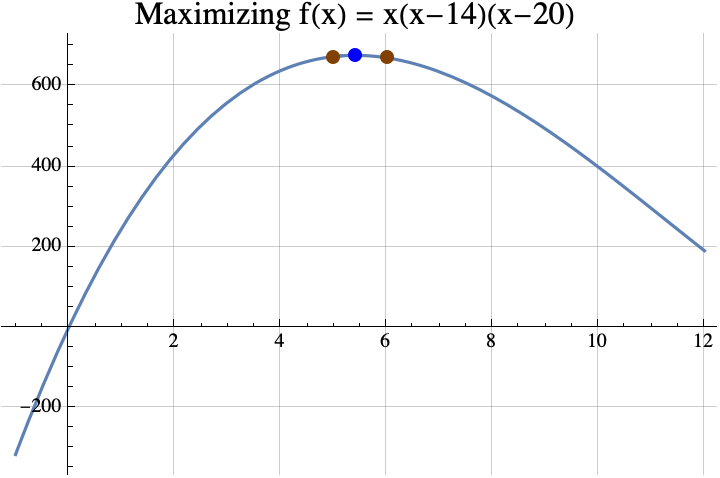Finding the maximum among reals is easy if you know differential calculus and the quadratic formula.  According to differential calculus, the maximum can only occur if the “derivative” of $f(x)$ is zero.   $$f(x) = x (x-14) (x-20) =x^3-34 x^2 + 280x.$$ The derivative of a polynomial can be found by multiplying the coefficient by the exponent and decreasing the exponent by 1. The result is $$f'(x) = 3 x^2 -68 x + 280.$$ If we put that in the quadratic formula, we get $$x=\frac{68\pm\sqrt{68^2 – 4 \cdot 3 \cdot 280}}{2 \cdot 3} = \frac{68\pm\sqrt{1264}}{6}\approx 5.41\ \mathrm{or\ } 17.26.$$ It turns out that 17.26 is a local minimum, so the local maximum occurs when $x\approx 5.41$.  According to the theorem, any integer $i$ that maximizes $f(i)$ must be within 1 of 5.41, so that integer can only be 5 or 6.  Now $f(5)= 675$ and $f(6) = 672$, so 5 is the integer that maximizes $f$.  Incidentally, $f(5.41) = 678.025021$ and the true maximum of $f(x)$ is $$f\left(\frac{68-\sqrt{1264}}{6}\right) = \frac{16}{27} \left(442+79 \sqrt{79}\right)\approx 678.025101610626.$$

We could actually use the theorem from part 1 to find the integer $i$ that maximizes $f(i)$.  Solving $f(x)=f(x+1)$ using the quadratic formula gives $$x=\frac{1}{6} \left(65\pm\sqrt{1261}\right).$$ If you replace the $\pm$ with the plus sign, you get the local minimum.  If you replace it with the minus sign, you get $$\frac{1}{6} \left(65-\sqrt{1261}\right)\approx 4.91.$$ According to the theorem from part 1, the integer that maximizes $f(x)$ must be round(4.91+1/2)=round(5.41)=5.  Often it is easier to find the maximum of $f$ rather than trying to solve $f(x)=f(x+1)$, so that is why the theorem above is useful.

If you want to see a more formal mathematical writeup, click here.  That PDF shows how you can generalize the three given theorems to a larger class of functions that are not necessarily strictly concave.  Also, in the last section of the paper, we sharpen the bound on the difference between the real maximum and the integer maximum.  Above, we showed that this difference was less than 1. If $f$ is continuously twice differentiable, then sharper bounds can be computed.

This concludes the three part series.

## Maximizing a function over integers (Part 2)

In part 1, we defined the term strictly concave and introduced the theorem that if $f$ is strictly concave and $f(x)=f(x+1)$, then the integer(s) that maximize $f$ are exactly the set $\{\mathrm{floor}(x+1), \mathrm{ceil}(x)\}$ which is usually a set containing only one number $\mathrm{round}(x+1/2)$.

### Parabolas

The simplest concave functions are parabolas with the vertex at the top.  All parabolas can be described by three real numbers $a$, $b$, and $c$, and the formula $y=a x^2 + b x + c$.  For strictly concave parabolas $a<0$.  For example, if $a=-2$, $b=3$, and $c=5$, then the function $y= f(x) = -2x^2 + 3 x +5$ is the parabola shown below.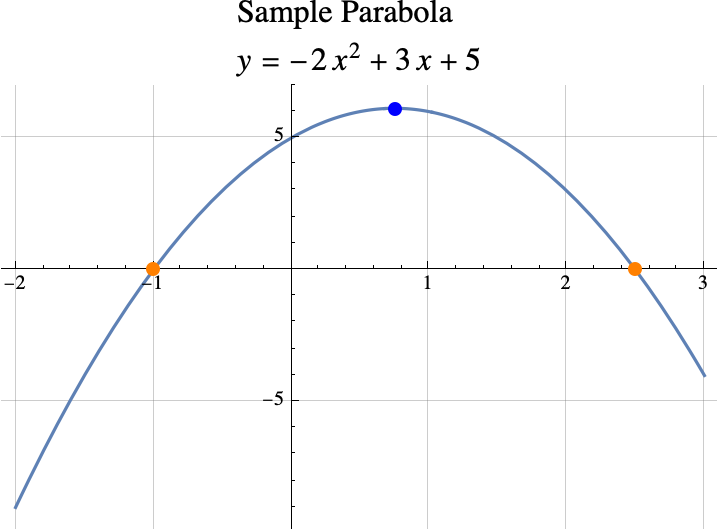If the vertex (shown above in blue) is above the x-axis, then the x-coordiante of the vertex can be computed by finding the points where the concave parabola crosses the x-axis (shown above in orange).  In our case, the parabola $y=f(x)$ crosses the x-axis when $0=y=f(x)$, or when \begin{aligned}0&= -2x^2 + 3 x +5\\0&=-(2x^2-3×-5)\\0&=-(2×-5)(x+1).\end{aligned}. If $0=\alpha\cdot \beta$, then either $\alpha=0$ or $\beta=0$.  So, the parabola crosses the x-axis when either $0=2×-5$ or $0=x+1$ which means that it crosses when $x=5/2$ or $x=-1$.  Parabolas are symmetric about the vertical line going through the vertex, so the x-coordinate of the vertex is half way between the x-coordinates of the crossing points (a.k.a the roots of $f(x)$).  \begin{aligned} x_\mathrm{vertex} &= \frac{x_\mathrm{crossing1} + x_\mathrm{crossing2}}2\\&= \frac{ -1 +5/2}2\\&=\frac{3/2}2\\&=3/4.\end{aligned}

So the x-coordinate of the vertex is $x=3/4$.  This is also the real number that maximizes $f(w)$ over all real numbers $w$.

In general, the x-coordinates of the crossing points can be found with the quadratic formula  $$x=\frac{-b\pm\sqrt{b^2-4ac}}{2 a}.$$ The  $\pm$ sign means that one crossing can be found by replacing $\pm$ with + and the other can be found by replacing the $\pm$ with -.  But, if you have one number that is $x_1=\alpha+\beta$ and another that is $x_2=\alpha-\beta$, then the average of the two numbers is just $\alpha$.  In other words if the two values of $x$ are $x=\alpha\pm\beta$, then the average value is just $\alpha$.  So, to computer the average x-coordinate of the crossing points, all we have to do is remove the $\pm$ sign and whatever it is applied to from the quadratic formula.  $$x_\mathrm{vertex} = \frac{-b}{2 a}.$$

### theorem #2

Informally, if $y=f(x)$ is a parabola, $f(x) = a x^2 + b x + c$, and $a<0$, then the integer(s) that maximize $f(x)$ are the set $\{\mathrm{floor}(x+1/2), \mathrm{ceil}(x-1/2)\}$ where $x=\frac{-b}{2 a}$.

If $x$ is an integer plus 1/2 (e.g. 2.5, 7.5, …), this set has two elements $x+1/2$ and $x-1/2$.  If $x$ is not an integer plus 1/2, the set has only one element $\mathrm{round}(x)$ and that is the integer that produces the highest possible value of $f(z)$ among all integers $z$.

### examples

Example 1: If $f(x)= -2x^2 + 3 x +5$, then $a=-2$, $b=3$, and $c=5$.  The values of $x$ in the theorem is the same as the x-coordinate of the vertex $$x=\frac{-b}{2a} = \frac{-3}{2\cdot(-2)}= \frac{-3}{-4}=3/4.$$ The integer(s) that maximize $f(z)$ among all integers $z$ are the set  \begin{aligned}\{\mathrm{floor}(x+1/2), \mathrm{ceil}(x-1/2)\}&= \{\mathrm{floor}(3/4+1/2), \mathrm{ceil}(3/4-1/2)\}\\&= \{\mathrm{floor}(5/4), \mathrm{ceil}(1/4)\}\\&=\{1\}.\end{aligned}

Example 2: If we lower the parabola from example 1 by a little bit setting $f(x)= -2x^2 + 3 x +\sqrt{17}$, then $a=-2$, $b=3$, and $c=\sqrt{17}$.  The value of $x$ in the theorem is the same as the x-coordinate of the vertex $x=\frac{-b}{2a} =3/4.$  The result does not depend on $c$, so as in example 1, the integer that maximizes $f(z)$ among all integers $z$ is $z=\mathrm{round}(3/4)=1$.

Example 3: $f(x)= -x^2+x = (1-x)x$, then $a=-1$, $b=1$, and $c=0$.  The value of $x$ in the theorem is the same as the x-coordinate of the vertex $$x=\frac{-b}{2a} =\frac{-1}{2\cdot (-1)}=1/2.$$ The integer(s) that maximize $f(z)$ among all integers $z$ are the set  \begin{aligned}\{\mathrm{floor}(x+1/2), \mathrm{ceil}(x-1/2)\}&= \{\mathrm{floor}(1/2+1/2), \mathrm{ceil}(1/2-1/2)\}\\&= \{\mathrm{floor}(1), \mathrm{ceil}(0)\}\\&=\{1,0\}.\end{aligned} The integers that maximize $f(z)$ among all integers $z$ are 0 and 1.  If we look at the graph of $f(x)$ below, we can see that the graph crosses the x-axis at $x=0$ and $x=1$.  So, $f(0)=f(1)=0$.  All other integers inputs produce negative values.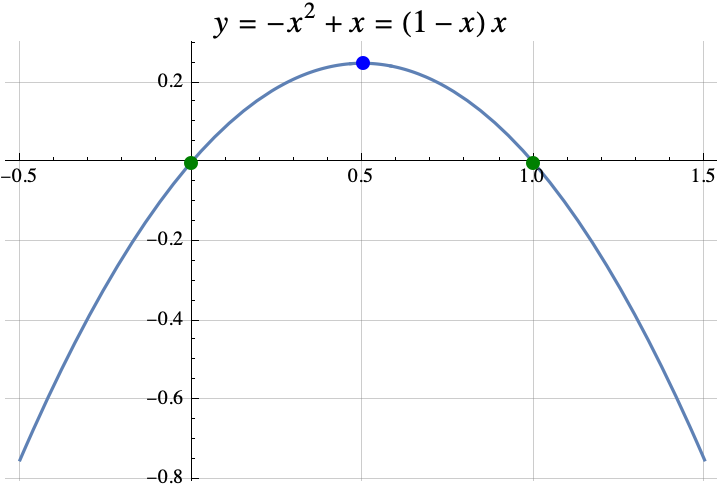### the spirit of the proof

It turns out that Theorem 2 can be proven from theorem 1.  Recall that in Theorem 1, we wanted to find the value of $x$ where $f(x)=f(x+1)$.  If we found that value, then the integer(s) that maximize $f$ are exactly the set $\{\mathrm{floor}(x+1), \mathrm{ceil}(x)\}$.  In Theorem 2, $f(x) = a x^2 + b x + 1$.  So if $f(x)=f(x+1)$, then \begin{aligned}a x^2 + b x + c &= a (x+1)^2 + b (x+1) + c\\a x^2 + b x &= a (x+1)^2 + b (x+1)\\ a x^2 + b x &= a (x^2+2x+1)+ b x+b\\ a x^2 &= a x^2+2ax+a+ b \\ 0 &= 2ax+a+ b \\ -a-b &= 2ax \\ \frac{-a-b}{2a} &= x\\ \frac{-b}{2a}-\frac12 &= x\\\end{aligned}.

Thus the integers that maximize $f(x)$ must be \begin{aligned} \{\mathrm{floor}(x+1), \mathrm{ceil}(x)\ \}&= \{\mathrm{floor}(\frac{-b}{2a}-\frac12+1), \mathrm{ceil}(\frac{-b}{2a}-\frac12)\} \\ &= \{\mathrm{floor}(\frac{-b}{2a}+\frac12), \mathrm{ceil}(\frac{-b}{2a}-\frac12)\}.\end{aligned}

### Why DId I WANTED to know this

I was looking at several games where the optimal strategy depended on finding an integer $t$ that maximized $f(t)$.  In the game Slay the Spire, I wanted to maximize the amount of poison damage that I was going to do with the cards “Noxious Fumes” and “Catalyst”.  If I played the “Catalyst” on turn $t$ and the combat ended on turn $T$, then the poison damage done was $$\frac{(t+1)t}{2} -1 + (T-t)t$$ where  $\frac{(t+1)t}{2}-1$ (note the triangle number) was the damage done by  “Noxious Fumes” and $(T-t)t$ was the additional damage done by playing the Catalyst.  I wanted to maximize $f(t) = (T-t)t = -t^2 + T t$.

Using Theorem 2, $a=-1$, $b=T$, and $c=0$.  The Theorem says that the maximum damage occurs when you play the “Catalyst” on round $t$ where $t$ is contained in the set $\{\mathrm{floor}(x+1/2), \mathrm{ceil}(x-1/2)\}$ with $x=\frac{-b}{2 a}$.  So $x=\frac{-T}{2\cdot(-1)}=T/2$.  The best time to play the Catalyst was around half way through the combat. The catalyst should be played on round $T/2$ if $T$ is even.  If $T$ is odd, then the best round to play the “Catalyst” was for $t$ in the set $$\{\mathrm{floor}(T/2+1/2), \mathrm{ceil}(T/2-1/2)\}= \{\frac{T+1}{2}, \frac{T-1}{2} \}.$$

### summary

So the first two theorems formalized two rules of thumb:

1. If you can find an $x$ where $f(x)=f(x+1)$, then the optimal integer(s) is $z=\mathrm{round}(x+1/2)$ with a special round that provides two answers if $x$ is an integer, and
2. If $f(x)=a x^2 + b x + c$ (a parabola), then the optimal integer(s) is $z=\mathrm{round}(x)$ where $x=\frac{-b}{2 a}$ which is the $x$ that maximizes $f(w)$ over all real numbers $w$ (i.e. the x-coordinate of the vertex).

If you want to see a more formal mathematical writeup, click here.

## Maximizing a function over integers (Part 1)

I proved a few simple but interesting theorems about integers that maximize a function $f$ when $f$ is a strictly concave function that maps real numbers to real numbers.  For example, the real number that maximizes $f(x)=x(1-x)$ is $x=1/2$, but among all the integers, the maximum possible value of the function is 0. And that maximum is achieved twice with integers 0 and 1, $f(0)=0=f(1)$.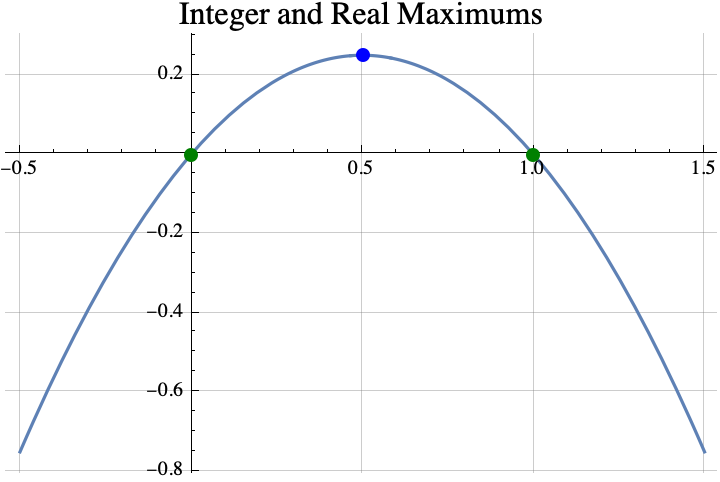A function is strictly concave if you can pick any two points on its graph, draw a line between them, and the curve $y=f(x)$ between the two points lies entirely above the line between the two points.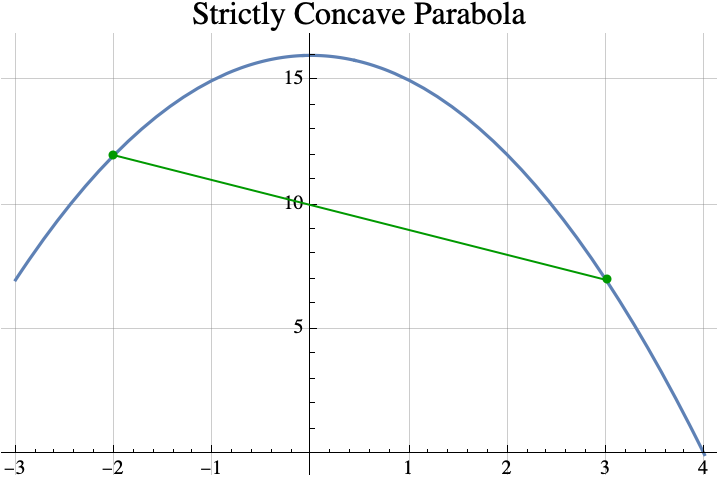### Theorem #1

Informally stated, the first theorem is that if you can find a real number $x$ such that $f(x)=f(x+1)$, then the integer(s) that maximize the concave function $f$ are the set $\{\mathrm{floor}(x+1), \mathrm{ceil}(x)\}$ where “floor” just means round down and “ceil” means round up.  That set usually contains only one element which is $\mathrm{round}(x+1/2)$, but if $x$ is an integer, then it will contain two consecutive integers $x$ and $x+1$.

For example, if $f(x) = 4-4x^2$, then the value of $x$ that satisfies $f(x)=f(x+1)$ is $x=-1/2$ because $f(-1/2)=3=f(1/2)$.  So the theorem says that any integer that maximizes $f$ must be in the set  \begin{aligned}\{\mathrm{floor}(x+1), \mathrm{ceil}(x)\} &= \{\mathrm{floor}(-1/2+1), \mathrm{ceil}(-1/2)\}\\&= \{\mathrm{floor}(1/2), \mathrm{ceil}(-1/2)\} \\&= \{0\}.\end{aligned} The integer that does the best is 0 which is also the real number that maximizes $f$.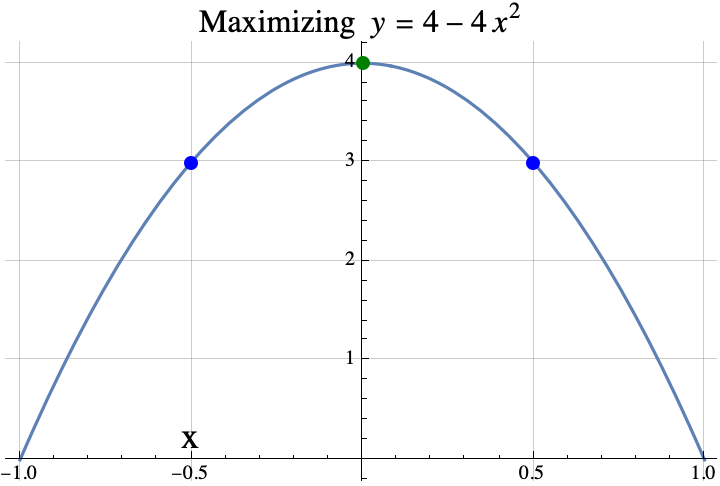Here is another example.  If $f(x) = \sin(x)$, then the value of $x$ that satisfies $f(x)=f(x+1)$ is $x=1.0708$ because $f(1.0708)=0.877583=f(2.0708)$.  So the theorem says that any integer that maximizes $f$ must be in the set  \begin{aligned}\{\mathrm{floor}(x+1), \mathrm{ceil}(x)\} &= \{\mathrm{floor}(1.0708+1), \mathrm{ceil}(1.0708)\}\\&= \{\mathrm{floor}(2.0708), \mathrm{ceil}(1.0708)\} \\&= \{2\}.\end{aligned}.  The integer that does the best is 2.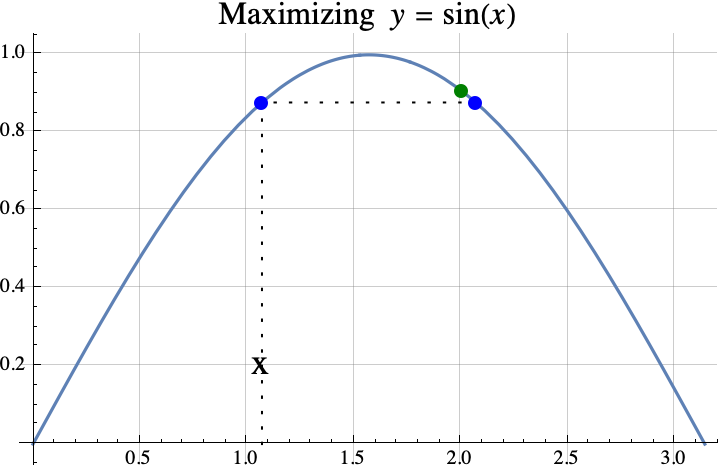So, that was the first theorem.  In part 2, we state a second theorem about finding the integer which maximizes a quadratic with some examples and one application the game “Slay the Spire”.

If you want to see a more formal mathematical writeup, click here.

## “Category Theory for Programmers”

I’ve been reading “Category Theory for Programmers” which was suggested to me by Mark Ettinger.  This book presents many examples in C++ and Haskell.  It teaches you some Haskell as you read the book.  It uses almost zero upper level mathematics and it skips almost all of the mathematical formalities.  If you decide that you want to read it, then you might want to read the first six chapters of “Learn You a Haskell for Great Good!” and write a few small Haskell programs first.  (I also would suggest trying to solve the first three problems in Project Euler https://projecteuler.net/archives  using Haskell.)

I find the book to be easy to read and informative.  When the author makes a statement like   A*(B+C) = A*B + A*C where * means categorical product, + means coproduct, and = means isomorphic, I find myself trying to figure out the categories where the statement is true and the categories for which it is false.  (It is true for the Category of Set and the Category Hask.  The book is mostly about those categories.) That type of thinking improves my understanding of category theory.  The book is also reawakening the parts of my brain that had forgotten parts of category theory and Haskell.

Interestingly, in category theory, $A*(B+C) = A*B + A*C$ can be translated into the following theorems :

1.  A*(B+C) = A*B + A*C  is true for all positive integers A,B, and C,
2. max(A, min(B,C)) = min( max(A,B), max(A,C))  for all real numbers A, B, and C,
3. lcm(A, gcd(B,C)) = gcd( lcm(A,B), lcm(A,C) )   where  lcm means least common multiple and gcd means greatest common denominator, and
4. intersection(A, union(B,C)) = union( intersection(A,B), intersection(A, C)).
If you don’t believe the four theorems, here is some Mathematica Code which tests each theorem:
Unprotect[C];
test[ funcRandChoose_, prod_, sum_, i_] := Tally[ Table[
A = funcRandChoose[];
B = funcRandChoose[];
C = funcRandChoose[];
prod[ A, sum[B, C]] == sum[ prod[A, B] , prod[A, C]],
{i}]];

test[ RandomInteger[{1, 1000}] &, Times, Plus, 100]
test[ RandomInteger[{-1000, 1000}] &, Max, Min, 100]
test[ RandomInteger[{-1000, 1000}] &, LCM, GCD, 100]
test[ RandomSample[ Subsets[ Range]] &, Intersection, Union, 100]

« Older entries